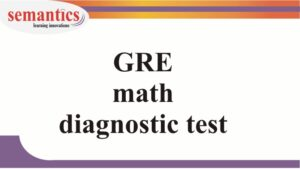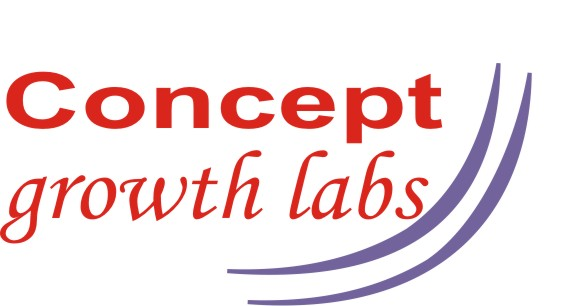GRE Math diagnostic testTake this diagnostic test and let us know your scores.

We can have a counselling session based on this data.

Book your counselling session by filling this formGRE Math Diagnostic test

Duration 10 mins

1 / 5

Compare quantity A and quantity B using additional information centered above the two quantities (if such information is given) and select one of the following answer choices.

st = sqrt(10)

Quantity A: S2

Quantity B: 10/t2

2 / 5

Compare quantity A and quantity B using additional information centered above the two quantities (if such information is given) and select one of the following answer choices.

x<y<z

Quantity A: (x+y+z)/3

Quantity B: y

3 / 5

Select one or more answer choices

Which of the following numbers is/are multiples of 2,4 and 6?

4 / 5

Select one or more answer choices

Which of the following digits could be the unit digit of 43n where n is a positive integer? Indicate all such answers?

5 / 5

Enter the correct value

A retailer buys a dozen pens for Rs.90. The wholesaler gives him a pen free on the condition that he should sell at Rs.9 per pen. What will be the retailer’s percentage profit?

0%

What next?

Do a Text Completion diagnostic test here

Do a Long Reading Comprehension passages diagnostic test here

Do a Short Reading Comprehension passages diagnostic test here